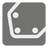Convex Hull
Simple
RU English ES

You are given a list of points on a coordinate plane. We need you find the convex hull formed by these points. The convex hull of a set X of points in the Euclidean plane is the smallest convex set that contains X. For instance: when X is a bounded subset of the plane, the convex hull may be visualized as the shape formed by a rubber band stretched around X. If a point lies on edge, it's included.

The points are presented as a list of coordinates [x, y] in which x and y are integers. The result returns as a sequence of indexes of points in the given list; points lie on the convex hull in clockwise order (see the picture). The sequence starts from the bottom...

You should be an authorized user in order to see the full description and start solving this mission.